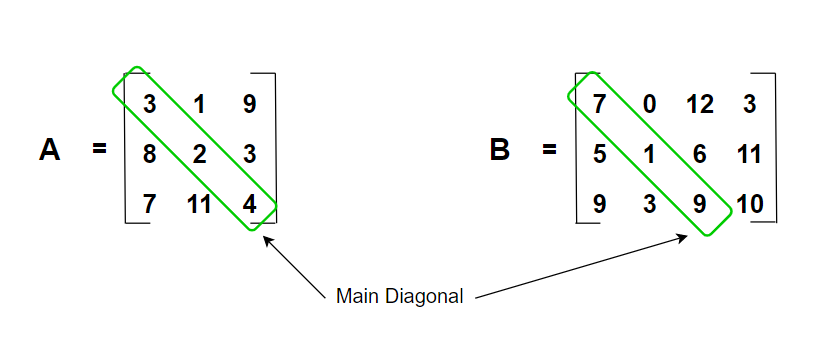# Extract Values Above Main Diagonal of a Matrix in R

• Last Updated : 22 Jun, 2022

In this article, we are going to know how to extract the values above the main diagonal of a matrix in the R programming language.

The main diagonal of a matrix is a straight path that connects the entries (or elements) in a matrix whose row and column are the same. The number of elements in the main diagonal is equivalent to either the number of rows or columns in the matrix. In matrix A, for every row i, and every column j of the matrix, the diagonal elements of the matrix are given by the following :

`Aij where i=j````Example:
Input Matrix : [[3, 1, 9],
[8, 2, 3],
[7, 11, 4]]
Output : 1, 9, 3
Explanation : In the above matrix the main diagonal is [3, 2, 4]
and we have to extract values above main diagonal elements.
so, the answer will be 1, 9, 3```

## Creating simple matrix

The matrix can be created using the matrix() method which is used to arrange the specified data elements into the specified number of rows and columns. The method has the following syntax :

Syntax : matrix (data, nrow = rows, ncol = cols)

Parameters :

• data – The data to be arranged into the matrix.
• rows – The number of rows in matrix.
• cols – The number of columns in matrix.

## R

 `# declaring the number of rows``nrow <- 4`` ` `# declaring the number of columns``ncol <- 4`` ` `# creating matrix``mat <- ``matrix``(1:16, nrow = nrow, ``              ``ncol = ncol)`` ` `# printing thr matrix ``print ``(``"Matrix : "``)``print``(mat)`

Output

``` "Matrix : "
[,1] [,2] [,3] [,4]
[1,]    1    5    9   13
[2,]    2    6   10   14
[3,]    3    7   11   15
[4,]    4    8   12   16```

## Extracting the above values of the main diagonal in a matrix

A nested for loop can be used to iterate over rows and columns of the matrix. An outer loop is used to iterate over the row elements and an inner loop is used to iterate over the column elements. And if the condition is used to check if the column number is greater than the row number. The element is then extracted from the matrix.

## R

 `# declaring the number of rows``nrow <- 4`` ` `# declaring the number of columns``ncol <- 4`` ` `# creating matrix``mat <- ``matrix``(1:16, nrow = nrow, ``              ``ncol = ncol)`` ` `# printing thr matrix ``print ``(``"Matrix : "``)``print``(mat)`` ` `# getting the elements above``# the main diagonal elements``# looping over rows``for``(row ``in` `1:``nrow``(mat)) ``{``   ` `  ``# ooping over columns``  ``for``(col ``in` `1:``ncol``(mat)) ``  ``{``    ``# if column number is greater than row ``      ``if``(col > row)``      ``{``        ``# printing the element of the matrix``        ``print``(mat[row,col])``      ``}``  ``}``}`

Output

``` "Matrix : "
[,1] [,2] [,3] [,4]
[1,]    1    5    9   13
[2,]    2    6   10   14
[3,]    3    7   11   15
[4,]    4    8   12   16

A
 5
 9
 13
 10
 14
 15```

My Personal Notes arrow_drop_up